# R语言非参数模型厘定保险费率：局部回归、广义相加模型GAM、样条回归# 原文链接: http://tecdat.cn/?p=14121

• ### 保费没有细分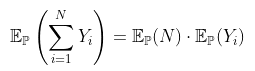Deviance Residuals:Min       1Q   Median       3Q      Max-0.5033  -0.3719  -0.2588  -0.1376  13.2700Coefficients:Estimate Std. Error z value Pr(>|z|)(Intercept)  -2.6201     0.0228  -114.9   <2e-16 ***---Signif. codes:  0 ‘***’ 0.001 ‘**’ 0.01 ‘*’ 0.05 ‘.’ 0.1 ‘ ’ 1(Dispersion parameter for poisson family taken to be 1)Null deviance: 12680  on 49999  degrees of freedomResidual deviance: 12680  on 49999  degrees of freedomAIC: 16353Number of Fisher Scoring iterations: 6> exp(coefficients(regglm0))(Intercept)0.07279295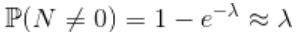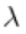可以解释为没有索赔的可能性。让我们将其可视化为驾驶员年龄的函数，

> plot(a,yp0,type="l",ylim=c(.03,.12))> segments(a[k],yp1[k],a[k],yp2[k],col="red",lwd=3)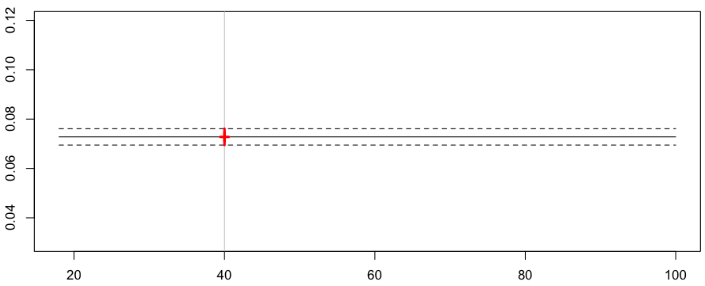> cat("Frequency =",yp0[k]," confidence interval",yp1[k],yp2[k])Frequency = 0.07279295  confidence interval 0.07611196 0.06947393

• （标准）泊松回归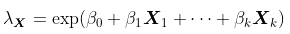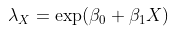> plot(a,yp0,type="l",ylim=c(.03,.12))> abline(v=40,col="grey")> lines(a,yp1,lty=2)> lines(a,yp2,lty=2)> points(a[k],yp0[k],pch=3,lwd=3,col="red")> segments(a[k],yp1[k],a[k],yp2[k],col="red",lwd=3)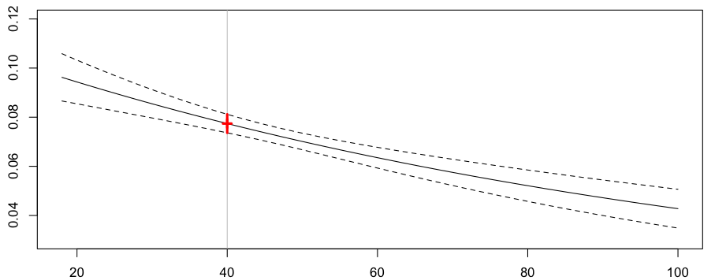> cat("Frequency =",yp0[k]," confidence interval",yp1[k],yp2[k])Frequency = 0.07740574  confidence interval 0.08117512 0.07363636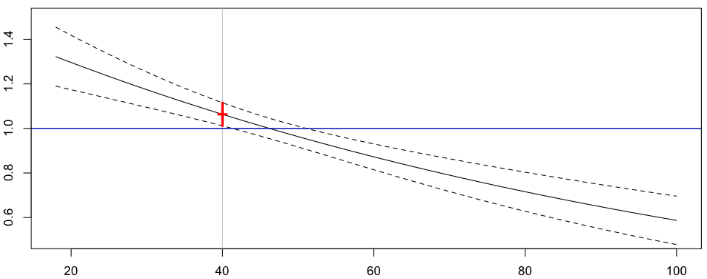• 纯非参数模型

> plot(a0,yp0,type="l",ylim=c(.03,.12))> abline(v=40,col="grey")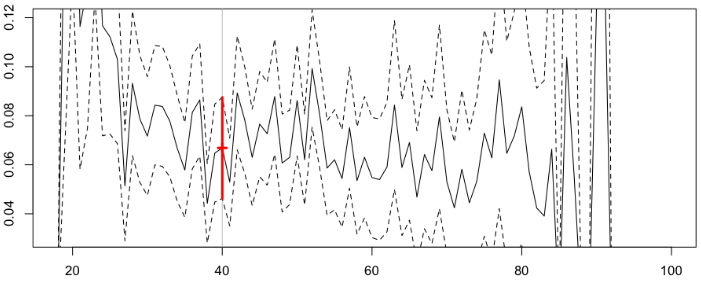Frequency = 0.06686658  confidence interval 0.08750205 0.0462311

> diff(log(yp0[23:25]))24         25-0.2330241  0.5223478

• 使用年龄段

> summary(regglmc1)Coefficients:Estimate Std. Error z value Pr(>|z|)(Intercept)                         -1.6036     0.1741  -9.212  < 2e-16 ***cut(ageconducteur, level1)(20,25]   -0.4200     0.1948  -2.157   0.0310 *cut(ageconducteur, level1)(25,30]   -0.9378     0.1903  -4.927 8.33e-07 ***cut(ageconducteur, level1)(30,35]   -1.0030     0.1869  -5.367 8.02e-08 ***cut(ageconducteur, level1)(35,40]   -1.0779     0.1866  -5.776 7.65e-09 ***cut(ageconducteur, level1)(40,45]   -1.0264     0.1858  -5.526 3.28e-08 ***cut(ageconducteur, level1)(45,50]   -0.9978     0.1856  -5.377 7.58e-08 ***cut(ageconducteur, level1)(50,55]   -1.0137     0.1855  -5.464 4.65e-08 ***cut(ageconducteur, level1)(55,60]   -1.2036     0.1939  -6.207 5.40e-10 ***cut(ageconducteur, level1)(60,65]   -1.1411     0.2008  -5.684 1.31e-08 ***cut(ageconducteur, level1)(65,70]   -1.2114     0.2085  -5.811 6.22e-09 ***cut(ageconducteur, level1)(70,75]   -1.3285     0.2210  -6.012 1.83e-09 ***cut(ageconducteur, level1)(75,80]   -0.9814     0.2271  -4.321 1.55e-05 ***cut(ageconducteur, level1)(80,85]   -1.4782     0.3371  -4.385 1.16e-05 ***cut(ageconducteur, level1)(85,90]   -1.2120     0.5294  -2.289   0.0221 *cut(ageconducteur, level1)(90,95]   -0.9728     1.0150  -0.958   0.3379cut(ageconducteur, level1)(95,100] -11.4694   144.2817  -0.079   0.9366---Signif. codes:  0 ‘***’ 0.001 ‘**’ 0.01 ‘*’ 0.05 ‘.’ 0.1 ‘ ’ 1> lines(a,yp1,lty=2,type="s")> lines(a,yp2,lty=2,type="s")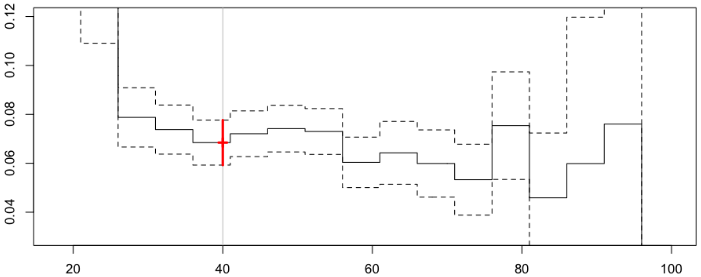Frequency = 0.0684573  confidence interval 0.07766717 0.05924742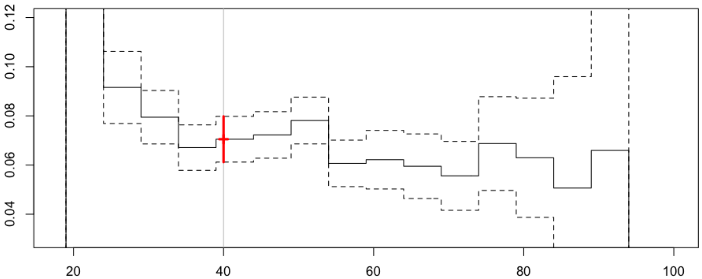Frequency = 0.07050614  confidence interval 0.07980422 0.06120807

• 移动平均

> value=40> h=5Frequency = 0.06913391  confidence interval 0.07535564 0.06291218

> value=40> h=5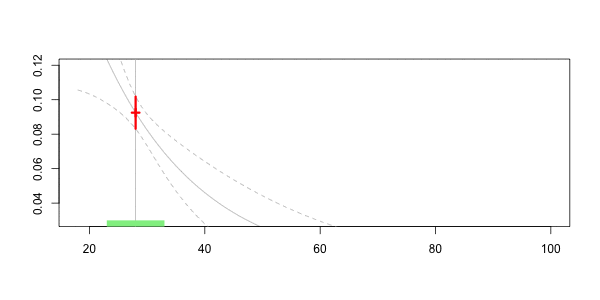Frequency = 0.07040464  confidence interval 0.07981521 0.06099408

• 样条平滑

> library(splines)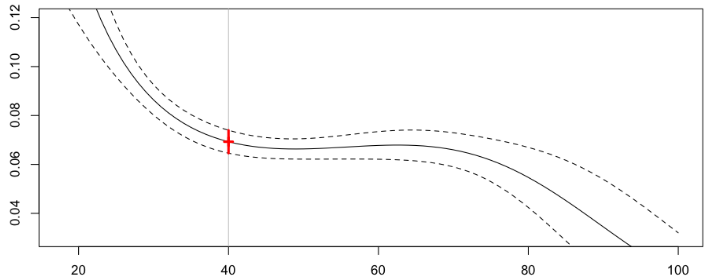Frequency = 0.06928169  confidence interval 0.07397124 0.06459215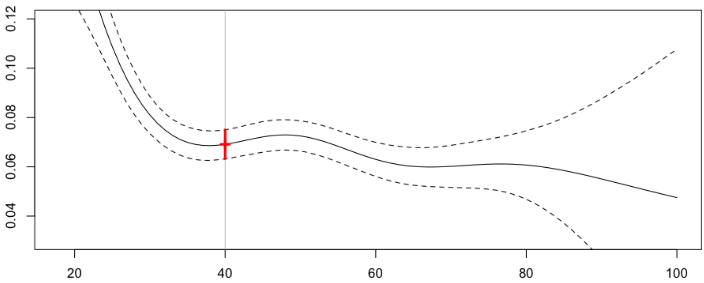Frequency = 0.06912683  confidence interval 0.07501663 0.06323702
• 不同模型的比较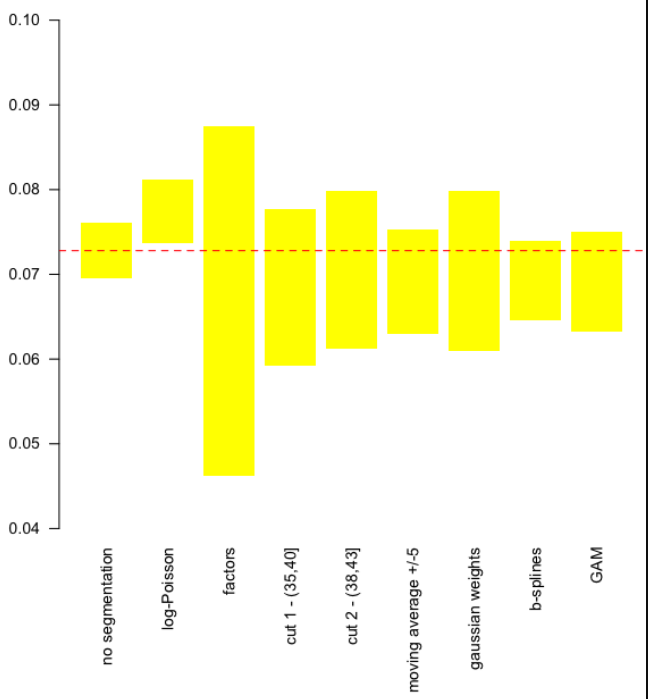https://wap.sciencenet.cn/blog-2674246-1243317.html

## 全部精选博文导读

GMT+8, 2021-10-19 17:35